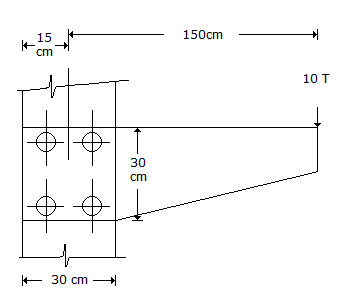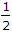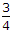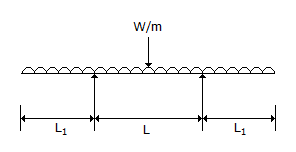# Civil Engineering - Strength of Materials

### Exercise :: Strength of Materials - Section 3

11.

If the width b and depth d of a beam simply supported with a central load are interchanged, the deflection at the centre of the beam will be changed in the ratio of

 A. b/d B. d/b C. (d/b)2 D. (b/d)2 E. (b/d)3.

Explanation:

No answer description available for this question. Let us discuss.

12.

In the given below figure, the rivets with maximum stress, are :A. 1 and 2 B. 1 and 3 C. 3 and 4 D. 2 and 4

Explanation:

No answer description available for this question. Let us discuss.

13.

The maximum stress intensity due to a suddenly applied load is x-times the stress intensity produced by the load of the same magnitude applied gradually. The value of x is

 A. 1 B. 2 C. 3 D.E.Explanation:

No answer description available for this question. Let us discuss.

14.

For the beam shown in below figure, the maximum positive bending moment is nearly equal to negative bending moment when L1 is equal toA. 1.0 L B. 0.7 L C. 0.5 L D. 0.35 L.

Explanation:

No answer description available for this question. Let us discuss.

15.

Strain energy of a member may be equated to

 A. average resistance x displacement B.stress x strain x area of its cross-section C.stress x strain x volume of the member D.(stress)2 x volume of the member + Young's modulus E.